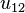## Tuesday, April 23, 2013

### Hearing Shape of the DrumMathematically ideal drums with membranes of these two different shapes (but otherwise identical) would sound the same, because the eigenfrequencies are all equal, so the timbral spectra would contain the same overtones. This example was constructed by Gordon, Webb and Wolpert. Notice that both polygons have the same area and perimeter.
To hear the shape of a drum is to infer information about the shape of the drumhead from the sound it makes, i.e., from the list of overtones, via the use of mathematical theory. "Can One Hear the Shape of a Drum?" was the title of an article by Mark Kac in the American Mathematical Monthly in 1966, but the phrasing of the title is due to Lipman Bers, and these questions can be traced back all the way to Hermann Weyl.

The frequencies at which a drumhead can vibrate depend on its shape. The Helmholtz equation tells us the frequencies if we know the shape. These frequencies are the eigenvalues of the Laplacian in the region. A central question is: can they tell us the shape if we know the frequencies? No other shape than a square vibrates at the same frequencies as a square. Is it possible for two different shapes to yield the same set of frequencies? Kac did not know the answer to that question.One of the possible modes of vibration of an idealized circular drum head (mode$u_{12}$ with the notation below). Other modes are shown at the bottom of the article.

Spectral geometry is a field in mathematics which concerns relationships between geometric structures of manifolds and spectra of canonically defined differential operators. The case of the Laplace–Beltrami operator on a closed Riemannian manifold has been most intensively studied, although other Laplace operators in differential geometry have also been examined. The field concerns itself with two kinds of questions: direct problems and inverse problems.

Inverse problems seek to identify features of the geometry from information about the eigenvalues of the Laplacian. One of the earliest results of this kind was due to Hermann Weyl who used David Hilbert's theory of integral equation in 1911 to show that the volume of a bounded domain in Euclidean space can be determined from the asymptotic behavior of the eigenvalues for the Dirichlet boundary value problem of the Laplace operator. This question is usually expressed as Can one hear the shape of a drum?", the popular phrase due to Mark Kac. A refinement of Weyl's asymptotic formula obtained by Pleijel and Minakshisundaram produces a series of local spectral invariants involving covariant differentiations of the curvature tensor, which can be used to establish spectral rigidity for a special class of manifolds. However as the example given by John Milnor tells us, the information of eigenvalues is not enough to determine the isometry class of a manifold (see isospectral). A general and systematic method due to Toshikazu Sunada gave rise to a veritable cottage industry of such examples which clarifies the phenomenon of isospectral manifolds.

Direct problems attempt to infer the behavior of the eigenvalues of a Riemannian manifold from knowledge of the geometry. The solutions to direct problems are typified by the Cheeger inequality which gives a relation between the first positive eigenvalue and an isoperimetric constant (the Cheeger constant). Many versions of the inequality have been established since Cheeger's work (by R. Brooks and P. Buser for instance).

1.